Optimal Pit Removal in Watershed and Stream Network Delineation

Annjanette Dodd

# December 8, 2000

Background

Grid digital elevation models (DEM's) are data structures in which the topographic elevation of each pixel is stored in a matrix node.  Grid DEM's are readily available from the Internet (1:24,000 scale from http://edcnts12.cr.usgs.gov/ned/ or 1:250,000 scale from http://edc.usgs.gov/nsdi/gendem.htm).  Two primary hydrologic quantities derived from DEM's are slopes and flow directions.  TARDEM is a suite of programs that can be utilized for the analysis of digital elevation data to determine hydrologic quantities of watersheds (for details go to http://www.engineering.usu.edu/dtarb/).  The steps involved are: (1) Pit filling corrections, (2) Computation of slopes and flow directions; (3) Computation of contributing area and specific catchment area and (4) Channel network extraction and computation of other quantities.

Here I will focus on the first and second steps only.  The 'flood' routine in TARDEM involves a process of filling pits in the elevation data.  Unnatural pits found in digital elevation data can affect the resulting flow directions determined from grid DEM's.  Pits, rare in natural topography, are grid elements or sets of grid elements surrounded by higher terrain that, in terms of the DEM, do not drain (Figure 1).  These arise from errors that result from the discrete nature of DEM's.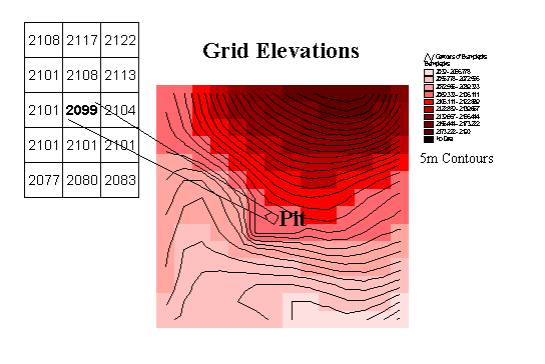Figure 1:  Example of an unnatural pit found in grid DEM's.

The 'flood' routine in TARDEM is an attempt to fill these unnatural pits by raising the elevation of each pit grid cell within the DEM to the elevation of the lowest pour point on the perimeter of the pit (Figure 2).  This is not an optimal method for determining the elevation of each pit grid cell.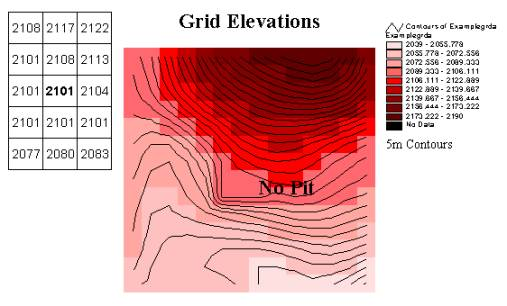Figure 2:  Example of pit removal using 'Flood' from TARDEM.

The 'D8' routine in TARDEM takes pit filled elevation data and creates flow directions using the eight-direction pour point model called the “D8 method”.  The direction of flow is from a pixel, determined by the steepest downward slope, to one of its eight neighbors, either adjacent or diagonally. The slope is computed as the difference in elevation between two adjacent cells divided by the distance between them. The direction is identified as one of eight flow directions starting due east and moving counter clockwise (Figure 3).  (In flat areas flow directions are assigned away from higher ground and towards lower ground using the method of Garbrecht and Martz).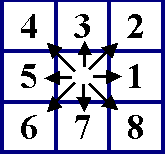Figure 3:  Eight flow directions utilized in the D8 method.

To improve the accuracy of flow direction along existing channel networks, the TARDEM includes the routine 'Streamtogrid' which formats EPA river reach files into grid DEM format and outputs a grid of flow directions along the rivers to enforce drainage along delineated river channels.

Statement of the Problem

In a large DEM grid, multiple pits can exist (Figure 4).  To better delineate flow paths, optimal pit removal (i.e., minimization of deviations from original elevations) should be performed.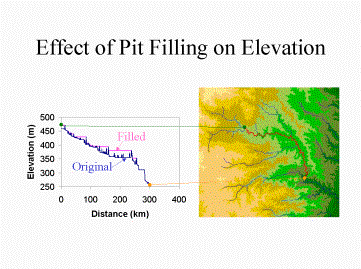Figure 4: Example of multiple pits in a large DEM. (Lecture presented by David Tarboton)

The purpose of this project is to create a program that minimizes the overall changes in elevation subject to grid drainage (determined by direction of steepest descent).   This will be accomplished by performing the following on a grid DEM with original elevations (ei):

1)   Fill the pits in the original elevation data using the program 'flood'

2)   Calculate the flow directions using the D8 method

The result is a grid of flow directions utilizing the pit filled elevation data (fd)

(This is a first estimate of flow directions in the channel network)

3)      Set up objective function and constraint set of the system and optimize

The constraint set utilizes the flow directions (fd) and original elevations (ei)

The result is a new elevation grid with the set of elevations (en)

4)   Calculate the flow directions using the D8 method on the new elevations

5)   Repeat steps 3 and 4 until a "no change" criterion between old and new elevations is met

The TARDEM software can be utilized to perform all of the above steps except the optimization.  The rest of this paper is devoted to this optimization problem.

The Optimization

For a DEM grid with n rows and m columns, the problem can be written as a linearly constrained quadratic programming problem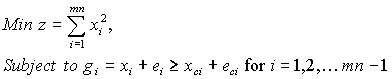(1)

where xi is the deviation from the original elevation at node i and ci represents the downstream node that node i drains into.  (There will actually be less than mn decision variables and mn-1 constraints when empty cells of the DEM exist.)  The new elevation for each node is determined by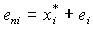. (2)

The constraint set, g, represents the imposed flow conditions determined using the D8 method on the old elevations.  The last node mn will not have a constraint because it will not have a pour point within the grid being analyzed.  The constraint set given in (1) can be written as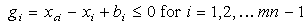(3)

where bi = eci - ei.  It is important to note that each constraint function is dependent only on the node it drains into.  Written in matrix form, with a coefficient matrix A and a right-hand side as a column vector representing the elevation differences of upstream and downstream pour nodes, the constraint set will have the form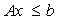.                                                                       (4)

Here A will be a (mn-1) x (mn) sparse matrix with a coefficient of –1 in column i, a coefficient of 1 in the column represented by the pour node ci, and zeros everywhere else.   The location of the pour node column (5) is only one of node i’s eight neighbors as determined from the D8 method.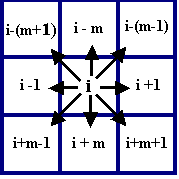Figure 5: Pour node identification

The coefficient matrix will be extremely large for large DEM's and will reduce computational efficiency.  Compressing the system is necessary for efficient optimization of large DEM's.  Separability of the problem in (1) can be exploited to reduce the size of the entire system.

Example 1:  Setting up the constraint set.

The 4 x 3 DEM grid (Figure 5a) will have the flow direction grid (Figure 5b) that results from the flooded grid (Figure 5c) (where the superscripts represent the node number i).  For the grid given in Figure 5a, the constraint set is summarized in Table 1 and an example of the coefficient matrix is given in Figure 6.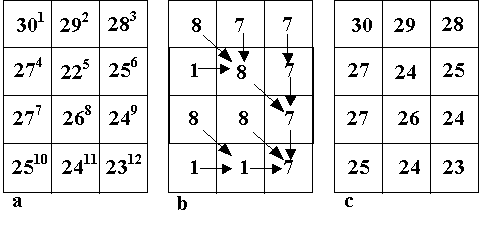Figure 5:  Example DEM grid pit filled using 'Flood' with flow directions determined using D8.

Table 1:  Constraint set for Example 1

 i xci - xi bi 1 x5-x1 -8 2 x5-x2 -7 3 x6-x3 -3 4 x5-x4 -5 5 x9-x5 2 6 x9-x6 -1 7 x11-x7 -3 8 x12-x8 -3 9 x12-x9 -1 10 x11-x10 -1 11 x12-x11 -1

 1 2 3 4 5 6 7 8 9 10 11 12 1 -1 0 0 0 1 0 0 0 0 0 0 0 2 0 -1 0 0 1 0 0 0 0 0 0 0 3 0 0 -1 0 0 1 0 0 0 0 0 0 4 0 0 0 -1 1 0 0 0 0 0 0 0 5 0 0 0 0 -1 0 0 0 1 0 0 0 6 0 0 0 0 0 -1 0 0 1 0 0 0 7 0 0 0 0 0 0 -1 0 0 0 1 0 8 0 0 0 0 0 0 0 -1 0 0 0 1 9 0 0 0 0 0 0 0 0 -1 0 0 1 10 0 0 0 0 0 0 0 0 0 -1 1 0 11 0 0 0 0 0 0 0 0 0 0 -1 1

Figure 6:  Constraint coefficient matrix, A, for Example 1.

Feasibility and Solution

The programming problem given in (1) has a constraint set that is closed, bounded, and non-empty.  The objective function is real-valued and continuous.  The Weierstrass and Local-global theorems guarantee that a solution exists for (1).  Since the constraints are linear, the feasible region is a convex set.  The Hessian of the objective function (matrix of second partial derivatives) is 2*I (I is the identity matrix) which is positive semi-definite, thus the objective function is convex.  The intersection of two convex sets is a convex set.  Therefore, any local solution is a global solution to the programming problem (1).  (Willis and Finney, 1998)

The development of the optimal solution begins by introducing a Lagrange multiplier, yi, for each constraint.  There are mn-1 constraints, thus there will be mn-1 Lagrange multipliers.  The Lagrangian for (1) is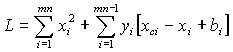.                                                                       (5)

Program (1) is a separable problem because each function can be written as a sum of functions, each depending on only one variable.  Due to separability of (1), the optimization problem decomposes into mn separate single-variable minimization problems or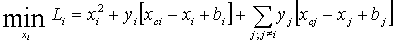.                                                                       (6)

Equation (6) is called the dual function and is maximized with respect to yi³0 for all i.  The last summation is over the Lagrange multipliers for which xcj = xi, i.e., for which xi is a downstream pour node.

The first order necessary conditions for a local maximum are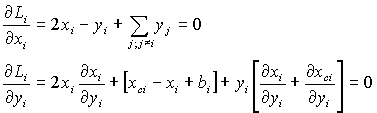(7)

Using the first necessary condition in (7), the optimal decision variables are given by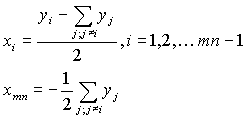(8)

The second necessary condition in (7) can be used to estimate yi.

If a node is not a downstream node for which any other nodes flow into, then (8) reduces to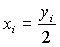.                                                                       (9)

Thus, if xi<0 then yi must equal zero to satisfy the non-negativity constraint for the Lagrange multipliers.  If a node is a downstream flow node and all yj for this node are zero (i.e., no other nodes pour into it) and xi<0, then yi=0.  For these nodes, the second necessary condition is automatically satisfied.  The remaining nsub decision variables that are not predetermined to be zero are used to determine the optimum for the system that satisfies (7).  This results in a system that is much reduced in size.

The original optimization problem in (1) is reduced to a sub-problem that involves optimizing a system of nsub decision variables.  For the nsub nodes that are left, the second necessary condition can be used to estimate yi or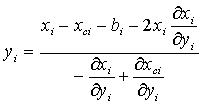(10)

where, from (8),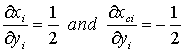(11)

Using an initial guess of y0=0, results, from (8), in x0=0.  From (10), with x0=0, the next value for yi is bi.  Thus, for the next set of yi³0, bi must be non-negative.  If bi£0, then, as discussed above, xi£0 and the next values for both xi and yi are zero.  Otherwise, yi=bi and the next xi can be evaluated from (8).  For each case that xi=0, the updated value will remain zero from step to step.  Thus, the problem becomes one of solving the original quadratic problem given in (1) with nsub decision variables and the corresponding constraints.  For the remaining yi’s to be a solution to original quadratic programming problem, then the following equality must hold (Belegundu & Chandrupatla)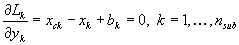.                                                                       (12)

where the corresponding xi’s are given by (8).  Therefore, utilizing separability, Lagrange multipliers, and principals of a quadratic programming problem, the original optimization problem reduces to the solution of a set of nsub linear equations that are easily solved.

Example 2: The system given in Example 1 is optimized using the method discussed above.       For the DEM given in Example 1 all of the xi's and yi's are predetermined to be zero except for x5, x9, and x12 (Table 2).  Thus, the resulting system to be solved is

Table 2:  Evaluation of Lagrange Multipliers in Example 2

 i xi bi 1 y1/2  Þ0 -8 2 y2/2  Þ0 -7 3 y3/2  Þ0 -3 4 y4/2  Þ0 -5 5 (y5-y1-y2-y4)/2      Þ y5/2 2 6 (y6-y3)/2  Þ y6/2  Þ0 -1 7 y7/2  Þ0 -3 8 y8/2  Þ0 -3 9 (y9-y5-y6)/2     Þ (y9-y5)/2 -1 10 y10/2  Þ0 -1 11 (y11-y7-y10)/2  Þ y11/2Þ0 -1 12 (-y8-y9-y11)/2      Þ -y9/2

Thus, the Lagrange multipliers can be determined from substitution into the two remaining constraint equations given in (12), or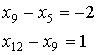Þ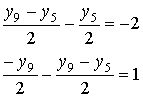Þ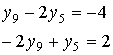Þ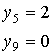Þ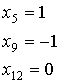The objective function evaluated for this solution is z=2.  The objective value from 'flood' was z=4.  It is important to note that if the objective function was set up to minimize the sum of the absolute values of the perturbations, both objective values would be the same.  Thus, for the linear programming problem, as given in this example, the solution would not be unique.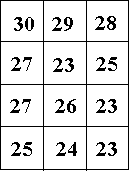Figure 7: Optimized DEM from Example 2.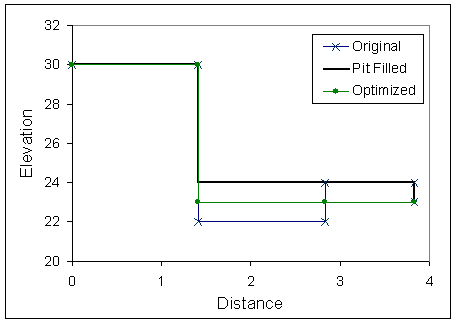Figure 8:  Profile of original pit and filled pit using optimization and 'Flood'

Discussion

The purpose of presenting the theory discussed here was an attempt to reduce the size of the original optimization problem for computational purposes.  Something is missing in the assumptions and derivations made above and further development of this theory must occur before a full scale computer program is developed to optimize the pit filling routine in large DEM's.

Example 3: Testing the above theory on a slightly larger grid with multiple pits.

 30 29 28 29 30 29 28 29 6 6 6 7 -8 -7 -6 -3 27 22 26 28 27 24 26 28 6 11 11 12 -5 2 -2 -2 27 26 24 26 27 26 24 26 14 14 15 16 -6 -5 -3 -3 25 21 21 23 25 23 23 23 14 15 19 20 -4 0 2 0 24 24 23 23 24 24 23 23 22 23 24 24 -2 -3 -3 -3 23 22 21 20 23 22 21 20 22 23 24 -1 -1 -1

a                                  b                                  c                                  d

Figure 9: a) Original Grid, b) Pit Filled Grid, c) Node number that cell pours into (ci), and d) grid representing bi's.

For this 4 x 6  DEM xi's and yi's are predetermined to be zero except for x6, x11, x15, x19, and x24 (Table 3).  Thus, the resulting system to be solved is

Table 3:  Evaluation of Lagrange Multipliers in Example 3.

 i xi bi 1-5,8-10,13, 17,18,21 yi/2  Þ0 negative 6 (y6-y1-y2-y3-y5)/2    Þ y6/2 2 7 (y7-y4)/2  Þ y7/2  Þ0 -2 11 (y11-y6-y7)/2     Þ (y11-y6)/2 -3 12 (y12-y8)/2      Þ y12/2  Þ0 -3 14 (y14-y9-y10-y13)/2  Þ y14/2 Þ0 0 15 (y15-y11-y14)/2     Þ (y15-y11)/2 2 16 (y16-y12)/2      Þ y16/2  Þ0 0 19 (y19-y15)/2     Þ (y19-y15)/2 -3 20 (y20-y16)/2      Þ y20/2  Þ0 -3 22 (y22-y17-y21)/2      Þ y22/2  Þ0 -1 23 (y23-y18-y22)/2      Þ y23/2  Þ0 -1 24 (-y19-y20-y23)/2      Þ -y19/2 -1

Thus, the Lagrange multipliers and optimal perturbations can be determined from substitution into the two remaining constraint equations given in (12), and are

 i yi xi 6 0 0 11 4 2 15 2 -1 19 4 1 24 -2

The optimized grid that results is

 30 29 28 29 27 22 26 28 27 26 26 26 25 21 20 23 24 24 24 23 23 22 21 18

Figure 10:  Optimized grid from Example 3

Obviously, the solution that is obtained in Example 3 does not satisfy the original flow directions as given in the constraint set and the pits are not removed.  In this example, not only are they not removed, the pits are not improved or are made worse (Figure 10).  This can only be a direct result of the assumptions made in eliminating Lagrange multipliers.  Based on the theoretical analysis discussed above, it seems that the nodes that should have been perturbed are nodes 6, 11,14,15, 19 and 24.  If node 14 is added into the constraint set, the perturbation at this node remains zero (because b14 = 0) and the system that results is inconsistent. This result indicates that a special constraint or condition is necessary when bi=0.

The results of Example 3 make it evident that further analysis of the theory and system composition is required to fully understand the interaction between the Lagrange multipliers and the constraints.

References

Belegundu, A. and Chandrupatla, T. (1999). Optimization Concepts and Applications in Engineering.  Prentice Hall, New Jersey.

Tarboton, D. http://www.engineering.usu.edu/dtarb/

Willis, R. and Finney, B. (1998). Environmental Systems Engineering. Dept. of Environmental Resources Engineering, Humboldt State University, California.